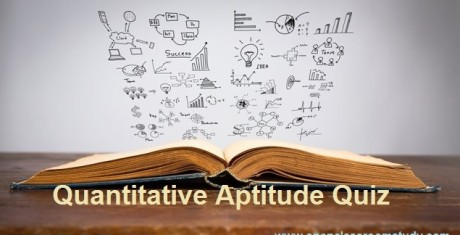# Mixed Aptitude Quiz - 6 : For IBPS PO Pre Exam 2016 (Part 4)#### Ques 1.

A man can row 12 km/hr in still water. When the river is running at 4 km/hr, it takes him 6 hrs to row to a place and come back. How far is the place?

Solution:
Time = Distance / Speed
D/(12+4) + D/(12-4) = 6
D/16 + D/8 = 6
D = 32 Km

#### Ques 2.

18, 96, 161, 213, 252, ?

Solution:
18+(13*6) = 96
96+(13*5) = 161
161+(13*4)= 213
213+(13*3)= 252
252+(13*2)= 278

#### Ques 3.

I.20x^2 – 31x + 12 = 0               II.20y^2 – y – 12 = 0

Solution:
20x^2 – 16x – 15x + 12 = 0
X = 4/5 and 3/4

20y^2 –16y+15y– 12 = 0
Y = 4/5 and -3/4

x = y or the relationship cannot be established.

#### Ques 4.

6209 + 3284 + 1927 + 2147 + 3228 + 3047 =?

Solution:
Add 1000 place digits = 6+3+1+2+3+3 = 18
18*1000 = 18000

Add 100  Place digits = 2+2+9+1+2+0 = 16
16*100 = 1600

Add 10 place digits = 0+8+2+4+2+4= 20
20*10 = 200

Add 1 place digits = 9+4+7+7+8+7 = 42

Now add = 18000+1600+200+42 = 19842

#### Ques 5.

Kishore lent out Rs.9500 at 8% per annum for a year 2 years 6 months.At the end of the duration,what amount he earned ?

Solution:
Rate * Time = 8*5/2 = 20%
100% ---- 9500
20%  ----    ?
Simple Interest  = 1900

#### Ques 6.

The ratio between the present age of P and Q is 7:4.The ratio between the age of P and Q four years ago is 2:1.What is the ratio age of P and Q four years hence

Solution:
Present age = 7:4 (difference 7-4=3)
4 years ago ratio = 2:1 (difference 2-1=1)

New Ratios:

Present age = 7:4 *1 = 7:4
4 years ago ratio = 2:1 *3 = 6:3

Difference between any person ratio = 1 units

Age difference = 4 years
P and Q  ages are 28 and 16
After 4 years Ratios = 32:20 = 8:5

#### Ques 7.

A cricketer has completed 14 innings and his average is 20.5 runs. How many runs must he make in his next innings so as to raise his average to 22?

Solution:
Total runs in 24 innings = 492
Total runs in 25 innings = 22*25 =550
25th innings score = 550-492 = 58 runs

#### Ques 8.

If the sides of a equilateral triangle is increased by 40% , 30%and 30%then a new triangle is formed. By what % perimeter of the triangle is increased?

Solution:
a = side of the triangle
Perimeter = 3a
New perimeter = a*140/100 + a*130/100 + a*130/100
= a(14+13+13)/10 = 40a/10 = 4a
% increased in perimeter = (4a – 3a/3a)*100
= 100/3 = 33.33%

#### Ques 9.

P and Q are two alloys of gold and copper in the ratio 5:3 and 3:7.Equal quantities of these alloy mixed to form a new alloy.The ratio of gold and copper in the new alloy is

Solution:
New ratio of P and Q = (5/8 + 3/10) / (3/8+ 7/10 )
P : Q = (25+12) : (15+28) = 37:43

#### Ques 10.

A , B and C invested Rs. 16000, Rs. 8000 and Rs. 16000 respectively to open a business. A left after 6 months. If after 8 months, there was a gain of Rs. 8010, then What will be the share of B?

Solution:
A : B : C = (16000 x 6) : (8000 x 8) : (16000 x 8)
è48 : 32 : 64 = 3 : 2 : 4

B’s share = Rs.8010 x 2/9 = Rs. 1780